GFG App
Open AppBrowser
Continue

Integration is a process of calculating the area of a particular region into many small strips and then calculate their area and sum it together. We know the formulas for calculating the areas of some standard shapes, integration allows us to calculate the area of any arbitrary region given the equation of its boundaries. Sometimes in more complex scenarios, we need to find the areas between the intersections of some curves. So we need to learn how to calculate the area between two curves. Let’s see how to solve such problems,

### Formula for Area between Curves

Let’s say we have two curves given in the figure below by f(x) and g(x). We know that f(x) ≥ g(x) in the interval [a, b]. Our goal is to find out the area bounded between two curves in the given interval. First, we find the points of intersections between two curves. The points of intersection are x = a and x = b. In the figure below, the shaded region gives the area bounded between two curves. We assume an elementary strip between the curves, the length of this strip is f(x) – g(x), and width is dx. So, the area bounded between two curves will be,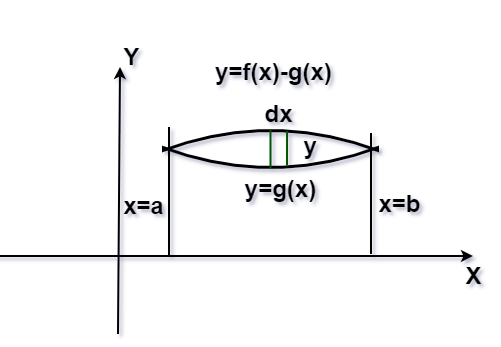In the previous formulation, we assumed that f(x) ≥ g(x) in the interval [a, b]. But it’s not always the case, let’s consider another case, f(x) ≥ g(x) in [a, c] and f(x) ≤ g(x) in [c, b], here a < c < b. So, the bounded area in this region is given in the figure below,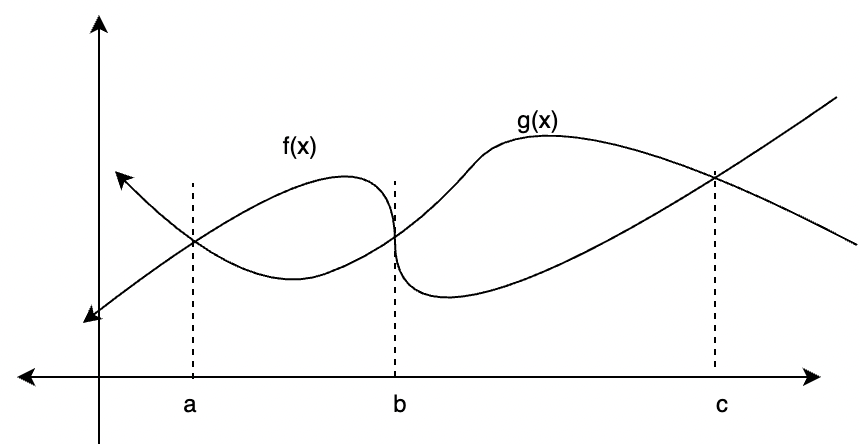Total Area = Area of PRQS + Area of QACB

=### Sample Problems

Question 1: Find the area bounded between two lines f(x) = 5x and g(x) = 3x from x =0 to x = 3.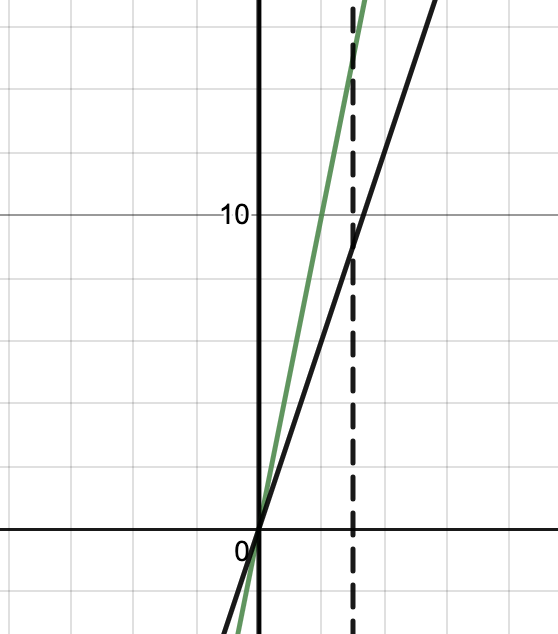Solution:

The figure below shows both the lines,

Figure

Area ====== 9

Question 2: Find the area bounded between two curves f(x) = x3 and g(x) = x2 between 0 and 1.

Solution:

The figure below shows both the curves, to find the bounded region, we first need to find the intersections.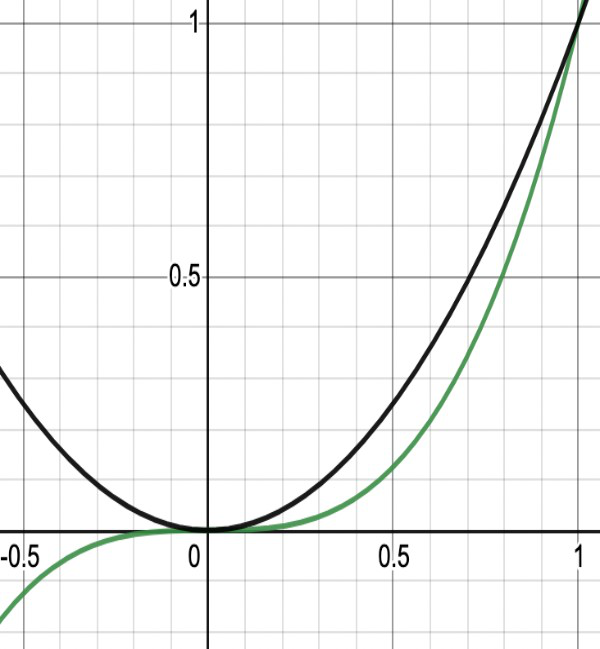f(x) = g(x)

⇒x3 = x2

⇒x2(x-1) = 0

⇒ x = 0 and 1

Figure

Area =======Question 3: Find the area bounded between the parabola y2 = 4x and x2 + y2 = 9.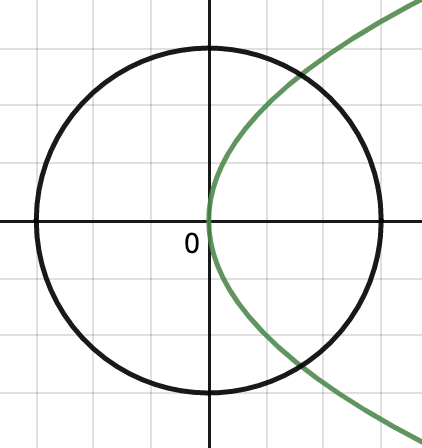Solution:

The figure below shows both the curves, to find the bounded region, we first need to find the intersections.

x2 + y2 = 12

Figure

Area =======Question 4: Find the area bounded between the parabola y2 = 4x, and its latus rectum.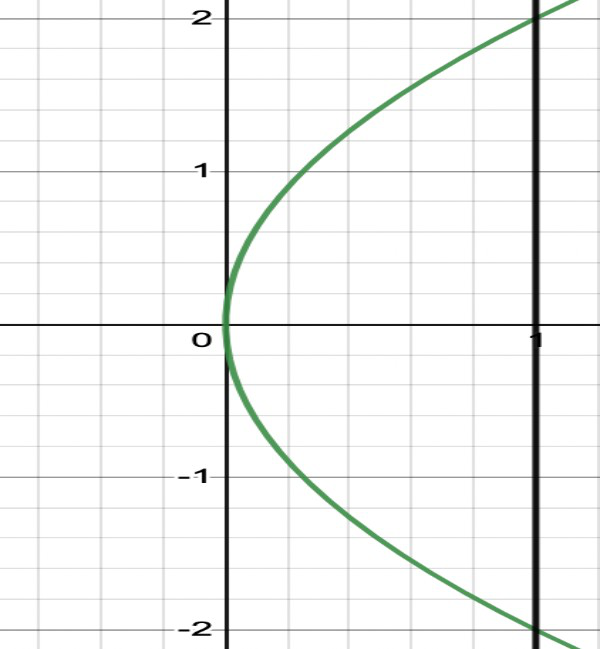Solution:

The figure below shows the parabola, and it’s latus rectum. Latus rectum is the line x = 1. We need to find the intersections,

y2 = 4

y = 2 and -2

Area = 2(Area of the region bounded by the parabola and x = 1 and x-axis in the first quadrant)

= 2(= 2= 4= 4==Question 5: The figure given below shows an ellipse 9x2+ y2 = 36 and a chord PQ. Find the area enclosed between the chord and the ellipse in the first quadrant.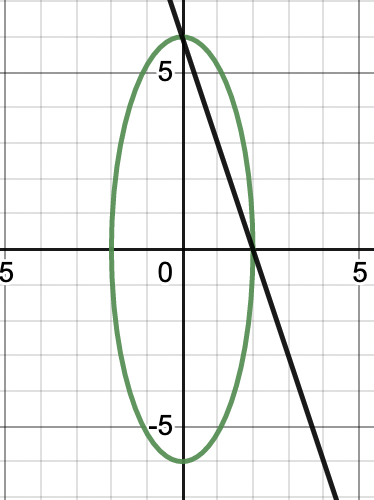Solution:

The equation of ellipse is,So, now the equation of the chord becomes,⇒ 3x + y = 6

⇒ y = 6 – 3x

So, now the required area will be.

A ====My Personal Notes arrow_drop_up